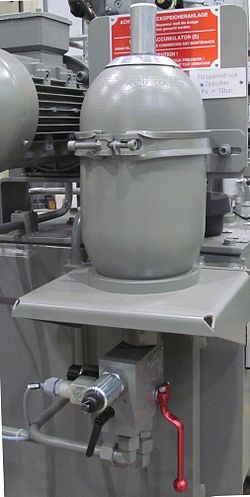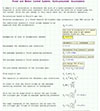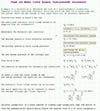## Hydro-Pneumatic AccumulatorsAccumulator image - Andy king50 [CC BY-SA 3.0] from Wikimedia Commons

Accumulators are used by mechanical engineers to store pressure energy in a hydraulic system. For most practical situations hydraulic fluids, usually mineral oil, used in hydraulic systems are incompressible, therefore engineers use the compressibility of a gas such as nitrogen to accumulate the hydraulic fluid.

Most accumulators consist of a spherical cylinder with a connection at one end for the hydraulic fluid and a connection at the other end for the gas. The gas and hydraulic fluid are kept apart by either a diaphragm or bladder made from elastomer that is a flexible elastic material.

Accumulators are installed within hydraulic systems for various reasons. These include energy accumulation, hydraulic fluid leakage compensation, shock absorption or as a damping element.

In order to calculate the correct size of accumulator to use for any application the gas law is used. For most applications it can be assumed the temperature will remain constant therefore a slightly modified version of Boyle’s law is used as shown:

The n value is included for consideration that there are two limiting values for the change in gas condition. The first is Isothermal, when the gas change takes place very slowly and therefore allows complete exchange of heat between the gas and its surroundings. In this condition n = 1. The second is Adiabatic, when the gas change is very rapid and there is no exchange of heat. In this condition n = 1.4.

The formula variables represent different volumes of gas and hydraulic fluid pressures that need to be considered:

V1 = Maximum gas volume of the accumulator, obtained from the manufacturers catalogue by estimating which size of accumulator is required.

V2 = The gas volume at P2

V3 = The gas volume at P3

P1 = The pre-charge pressure of the gas. For energy accumulation this should be about 90% of P2

P2 = The minimum system pressure required for the hydraulic fluid

P3 = The maximum operating pressure, somewhere between P2 and the maximum pump pressure of the hydraulic system, found by trial and error. The ratio between P1 and P3 should not exceed 4:1

To demonstrate how this works I have created a calculation sheet that shows how to calculate the hydraulic fluid available from a specified accumulator. Click on the image to display the calculation sheet.The calculation sheet above shows the method I used when I worked as a hydraulics design engineer. This method relies on guessing the size of accumulator, based on experience and then adjusting the initial guess once the calculation has been done to identify the correct size of accumulator. I have also made a 2nd version of the calculation sheet that demonstrates how Boyle’s law can be used without the need to make any initial guesses. Why not try both versions and see which version you prefer.These are live calculation sheets created in SMath Studio in the Cloud. You are free to change the input values online or alternatively if you have SMath Desktop you can download the sheet.